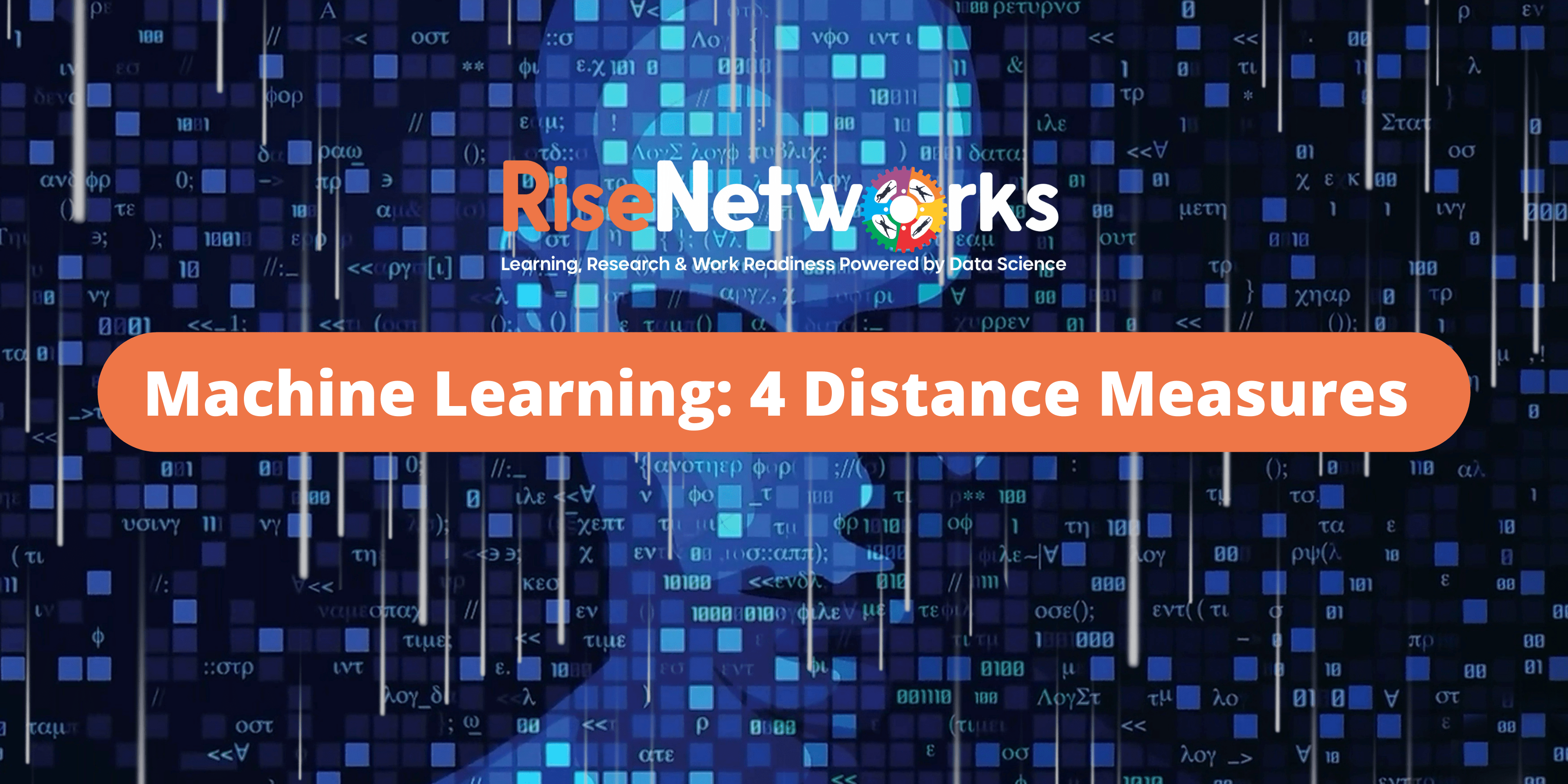Rise Networks# Machine Learning: 4 Distance Measures

Machine learning relies heavily on distance metrics.

Many popular and effective machine learning algorithms, such as k-nearest neighbours for supervised learning and k-means clustering for unsupervised learning, are built on top of them.

Depending on the type of data, different distance measurements must be chosen and employed. As a result, knowing how to construct and calculate a variety of popular distance measures, as well as the intuitions behind the resultant scores, is critical.

You will learn about distance measures in machine learning in this tutorial.

The Function of Distance Measures
Machine learning relies heavily on distance metrics.
The relative difference between two items in a problem area is summarized by a distance measure, which is an objective score.
Rows of data that describe a subject (such as a person, automobile, or house) or an event are the most common two objects (such as a purchase, a claim, or a diagnosis).

The most probable time you’ll come across distance measures is when you’re working with a machine learning algorithm that uses distance measures as its foundation. The k-nearest neighbour’s algorithm, or KNN for short, is the most well-known in this class.
The KNN method calculates the distance between fresh examples to provide a classification or regression prediction for them.

A shortlist of some of the more popular machine learning algorithms that use distance measures at their core is as follows:
K-Nearest Neighbors
Learning Vector Quantization (LVQ)
Self-Organizing Map (SOM)
K-Means Clustering
There are many kernel-based methods that may also be considered distance-based algorithms. Perhaps the most widely known kernel method is the support vector machine algorithm or SVM for short.
Do you know more algorithms that use distance measures?
Let me know in the comments below.

It is possible that various data types are utilized for different columns of the examples when computing the distance between two examples or rows of data. Real, boolean, category and ordinal values are all examples of values. It’s possible that different distance measures are needed for each, which are then added together to provide a single distance score.

The scales of numerical quantities can vary. It is typically a good practice to normalize or standardize numerical values before calculating the distance measure because this might have a significant impact on the calculation of the distance measure.

Numerical error in regression problems may also be considered a distance. For example, the error between the expected value and the predicted value is a one-dimensional distance measure that can be summed or averaged over all examples in a test set to give a total distance between the expected and predicted outcomes in the dataset. Once the spins are over the winnings shall be transferred to the bonus account and are subject to terms and situations win bola. Free spins online casino are exactly that, free spins. Besides that, their information and integrity additionally come to check. The calculation of the error, such as the mean squared error or mean absolute error, may resemble a standard distance measure.
As we can see, distance measures play an important role in machine learning. Perhaps four of the most commonly used distance measures in machine learning are as follows:
Hamming Distance
Euclidean Distance
Manhattan Distance
Minkowski Distance
What are some other distance measures you have used or heard of?
Let me know in the comments below.
You need to know how to calculate each of these distance measures when implementing algorithms from scratch and the intuition for what is being calculated when using algorithms that make use of these distance measures.

0